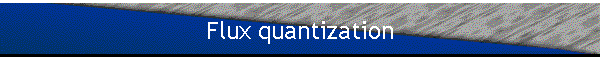If a charged particle travels in a field free region that surrounds another region, in which there is trapped magnetic flux F, then upon completing a closed loop the particle’s wave function will acquire an additional phase factor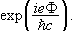But the wave function must be single valued at any point in space.  This can be accomplished if the magnetic flux F is quantized.  We need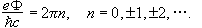This quantization of the magnetic flux is observed in superconductors.  Superconductivity is theorized to be due to a special correlation between pairs of electrons that extends over the whole body of the superconductor.  When a Type I superconductor is placed in a magnetic field and cooled below its critical temperature, it excludes all magnetic flux from its interior.  This is called the Meissner effect.  If there is a "hole" in the superconductor, then flux can be trapped in this hole.  The flux trapped in the hole must be quantized.  It has been experimentally verified that the trapped flux is quantized in units of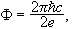thus verifying that the charge carriers in superconductors are indeed correlated electron pairs of charge 2e.

#### Magnetic monopoles

In Maxwell’s equations magnetic charges do not appear.  We have.  No magnetic charges have been confirmed to exist.  Quantum mechanics does not require that magnetic charges exist, but it unambiguously requires the quantization of magnetic monopoles and predicts the unit of magnetic charge if they should ever be found.

Assume that magnetic monopoles exist and that a magnetic monopole is located at the origin.  Then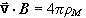and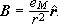We have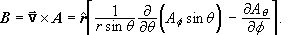A possible solution is A=eM(1-cosq)/(rsinq) in the f-direction.  But A is singular on the negative z-axis at q=p.  If we consider A just a device for obtaining B, then we can construct a pair of vector potentials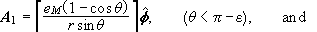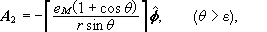which together yield the correct B everywhere.  A1 can be used everywhere except inside a cone defined by q=p-e around the negative z-axis, and A2 can be used everywhere except inside a cone defined by q=e around the positive z-axis.  In the overlap region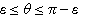either A1 or A2 can be used.  The two potentials lead to the same magnetic field and are therefore related to each other by a gauge transformation.The wave function of a charged particle depends on the particular gauge used.  In the overlap region we have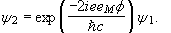Here e is the particle's electric charge.  The wave function must be single valued.  As we increase the azimuthal angle f from 0 to 2p, the wave function must return to its original value.  This is only possible if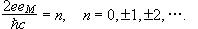We therefore find that eM must be quantized in units of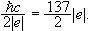The smallest magnetic charge possible is#### Summary:

Gauge transformations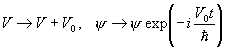,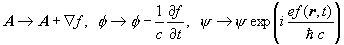,

change the Schroedinger equation and therefore change the wave function.  But they change both in such a way that the results of a measurement do not change.

However, knowing that the phase of the wave function depends on the gauge and the physical predictions do not depend on the gauge, we can reach some interesting conclusions.

• Magnetic Flux is quantized.
• The strength of magnetic monopoles must be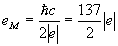.